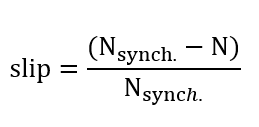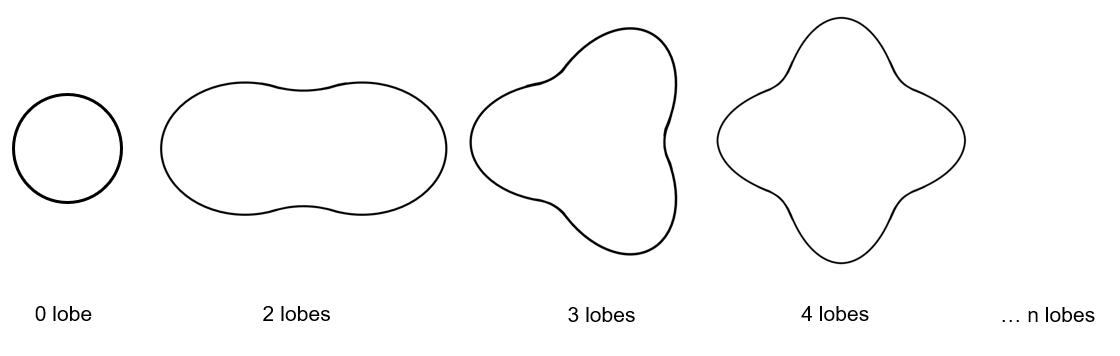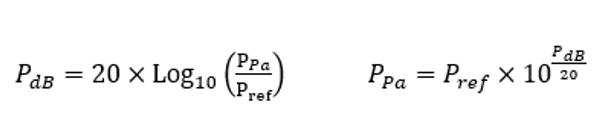# Inputs

## 1. Introduction

The total number of inputs is equal to 11.

Among these inputs, 3 are standard inputs and 8 are advanced inputs.

## 2.1 Line-Line voltage, rms

The rms value of the Line-Line voltage supplying the machine: “Line-Line voltage, rms” ( Line-Line voltage, rms value ) must be provided.

Note: The number of parallel paths and the winding connections are automatically considered in the results

## 2.2 Power supply frequency

The value of the power supply frequency of the machine: “ Power supply frequency ” ( Power supply frequency ) must be provided.

The power supply frequency is the electrical frequency applied at the terminals of the machine.

## 2.3 Definition mode

There are 2 common ways to define the working point. It can be defined by indicating the slip “ Slip ” or by defining the operating speed of the machine “ Speed ”.

## 2.4 Slip

When the choice of definition mode is “Slip” , the value of machine’s slip “Slip” ( Slip ) must be provided.

Note: The slip (s) corresponds to the difference between the speed of the rotating magnetic field in the stator (N s = Synchronous speed) and the rotor operating speed (N).

Unit can be % or PU.## 2.5 Speed

When the choice of definition mode is “Speed” , the value of operating speed “Speed” ( Speed ) must be provided.

## 3.1 Maximum engine order / No. Points per electrical period

Two kinds of inputs are possible:

Define the Max. engine order ( Maximum engine order ) or the No. points / elec. Period ( Number of points per electric period ).

When decomposing the Maxwell pressure, applied on the stator, to get its harmonic contributions, the “ max. engine order ” ( Maximum engine order ) is required to compute its decomposition in function of the time.

At a practical point of view, when the maximum engine order is equal to N, that leads to consider 2*N computation points over a complete rotation period of the rotor.

Note:
1. The input "Engine order" is in connection with the frequency of vibration.

"Engine order" refers to a mechanical revolution period of the motor whereas frequency refers to the considered electrical period.

Obviously, both are linked with speed.

For instance, sound power level can be displayed either by considering frequency or engine order.

2. There are two possibilities, either set an engine order or a number of points per electrical period.

For transient computations the minimum needed number of points per electrical period is 40.

So, when the engine order is not high enough to reach this constraint It is automatically modified to get 40 computation points per electrical period.

## 3.2 Maximum mode / spatial order

The “max. mode / spatial order ” ( Maximum mode / spatial order ) input allows the user to define the number of modes to be considered for the acoustic structural analysis. If the user selects 25, it means that the highest number of lobes in the stator deformation will be equal to 25 lobes. All deformations corresponding to more than 25 lobes will be dismissed.Number of lobes of the stator mechanical structure

## 3.3 Number of computations per tooth pitch

The “ No. comp./tooth pitch ” ( Number of computations per tooth pitch ) allows to choose the number of Maxwell pressure evaluations per tooth. The more points selected, the more accurate the Maxwell pressure harmonic decomposition will be.

## 3.4 Displayed pressure range (dB)

The “ displayed pressure range (dB) ” ( Displayed Maxwell pressure range ) is only related to the displaying of results.

It allows to increase/decrease the range of non-zero contributions displayed in the Maxwell pressure harmonic decomposition map.

When one chooses a displayed pressure range equal to 50 dB, only the contributions within the range of 50 dB and below the maximum computed value is displayed.

Note: The absolute reference value P ref (expressed in Pa), which corresponds to 0 dB, is indicated in the working point table.

Considering this referent value P ref , one can compute any Maxwell pressure value P Pa (expressed in Pa) from the ones expressed in Decibel P dB .

The relations between these quantities are illustrated below:The default value is equal to 60. The range of possible values is [20;100].

## 3.5 Number of rotor turns

This input allows us to define the number of rotor revolutions to consider the slip as far as possible.

Higher is the number of rotor revolutions better will be the results. However, this value has a huge impact on the computation time.

The default value is equal to 5. This is a good compromise between computation time and quality of results.

The variation range of values for this parameter is [1; 10].

## 3.6 Computation of forces on teeth

The “ computation of forces on teeth ” ( Additional analysis to compute the force on teeth ) allows to perform or not, the additional analysis which is the computation of force on teeth. Since this computation requires bigger memory and an additional computation time, this input allows to consider it as an additional option.

## 3.7 Mesh order

To get the results, the original computation is performed using a Finite Element Modeling.

Two levels of meshing can be considered for this finite element calculation: first order and second order.

This parameter influences the accuracy of results and the computation time.

By default, second order mesh is used.

## 3.8 Airgap mesh coefficient

The advanced user input “ Airgap mesh coefficient ” is a coefficient which adjusts the size of mesh elements inside the airgap. When the value of “ Airgap mesh coefficient ” decreases, the mesh elements get smaller, leading to a higher mesh density inside the airgap, increasing the computation accuracy.

The imposed Mesh Point (size of mesh elements touching points of the geometry) is described with the following parameters:

MeshPoint = (airgap) x (airgap mesh coefficient)

Airgap mesh coefficient is set to 1.5 by default.

The variation range of values for this parameter is [0.05; 2].

0.05 giving a very high mesh density and 2 giving a very coarse mesh density.

CAUTION:
Be aware, a very high mesh density does not always mean a better result quality.

However, this always leads to a huge number of nodes in the corresponding finite element model. So, it means a need of huge numerical memory and increases the computation time considerably.